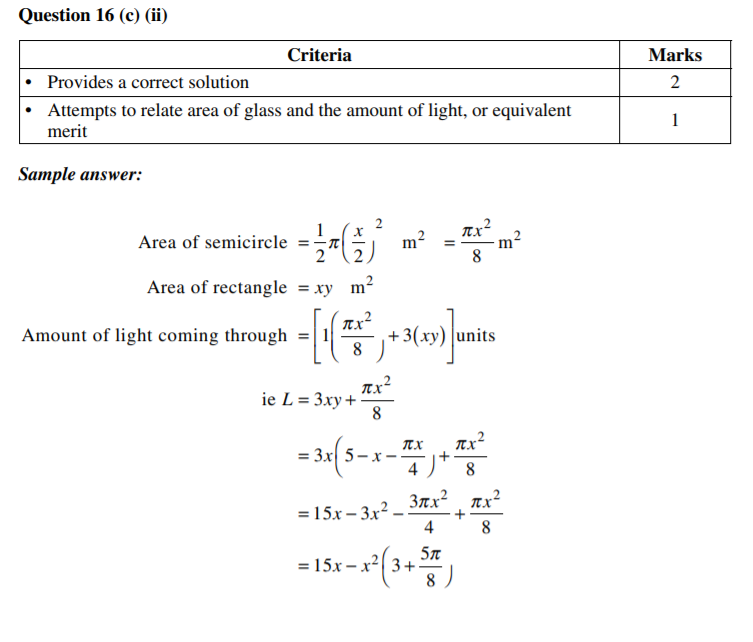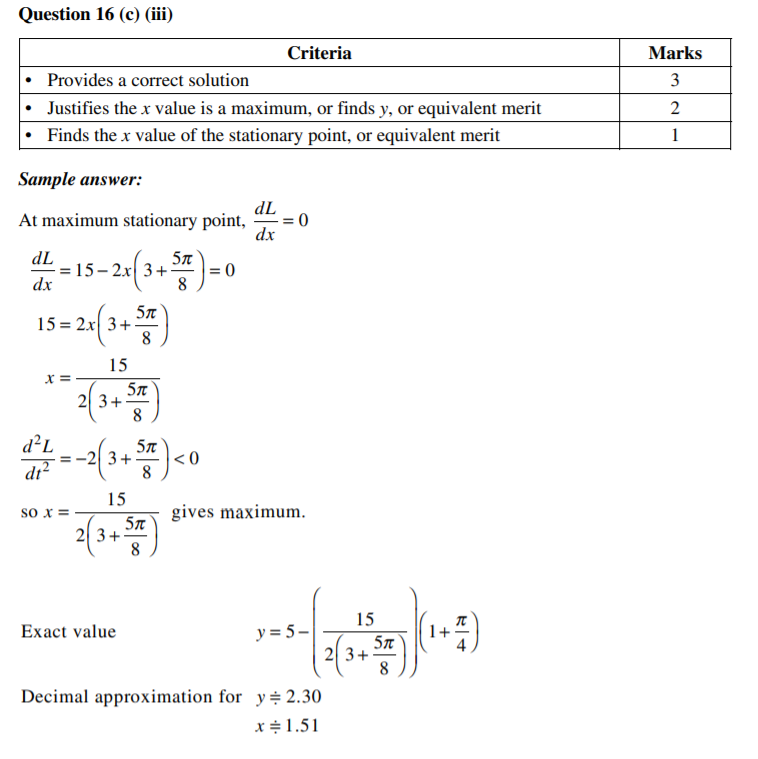# Max/ Min Optimization problem set

The following is a 25 mark test, this cover max/ min optimization problems – this should take 45 mins under exam conditions.

Questions.

1a) Two circles radii sum to 100 m. One circle has radius x m, show the area of both circles is represented by the equation below:

A = 2π(x2 – 100x + 5000)[2 marks]

b) For what value of x do these circles have the smallest area? [2 marks]

2) A soft drink company is designing their aluminium cans and want to optimize the greatest volume for the aluminium sheet of 200 cm2. What is the maximum volume this can be? [4 marks]

3) A square based prism shaped room for a house is being built, however, the painter wants the room to have every edge painted with a very expensive paint. What dimensions of the 300 mroom (including ceiling, walls, floor) will minimize the amount of expensive paint that must be applied to all of the edges. [5 marks]

From past HSC Questions:

2013 HSC Q14 B

2014 HSC Q 16C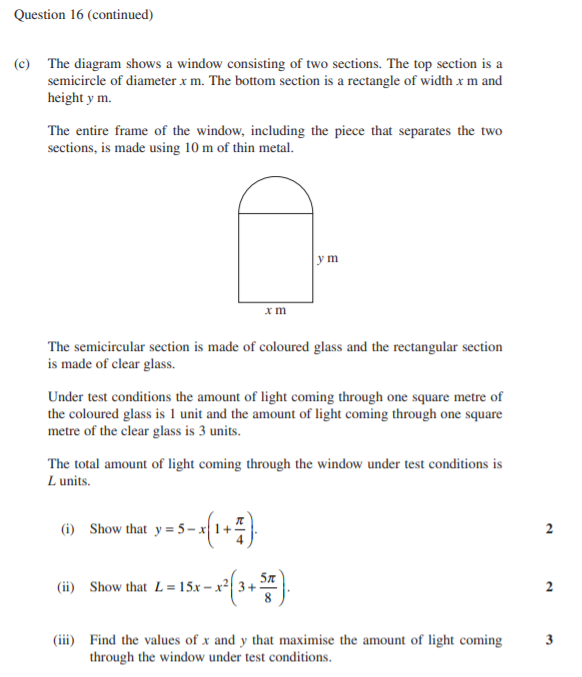4)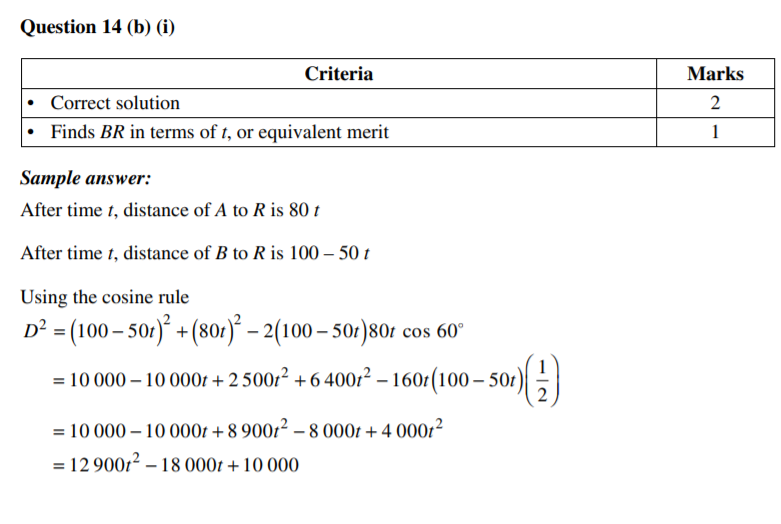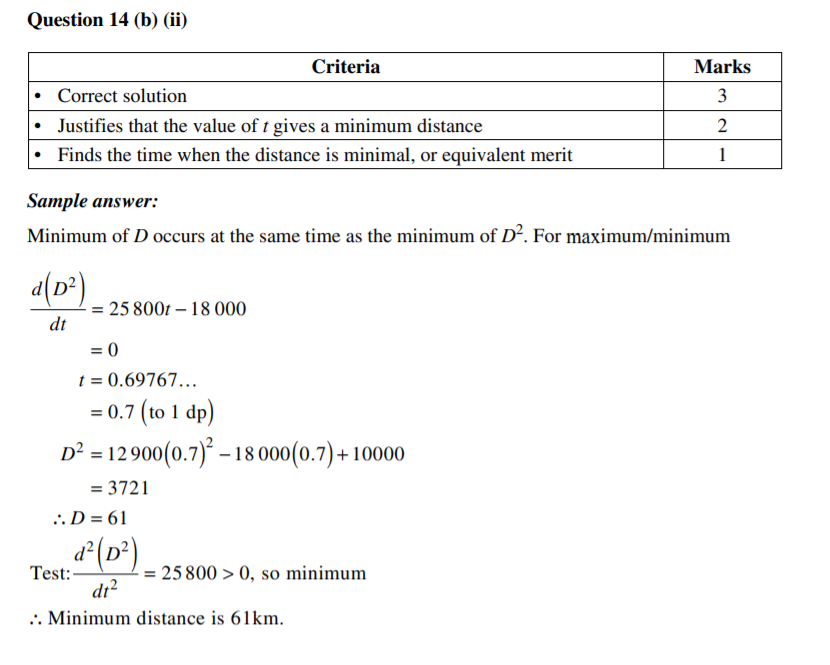5)